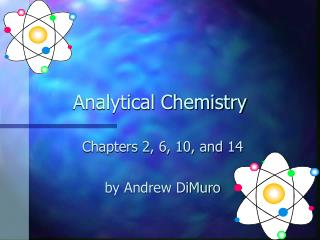DownloadDownload PresentationAnalytical Chemistry

# Analytical Chemistry

Télécharger la présentation## Analytical Chemistry

- - - - - - - - - - - - - - - - - - - - - - - - - - - E N D - - - - - - - - - - - - - - - - - - - - - - - - - - -
##### Presentation Transcript

1. Analytical Chemistry Chapters 2, 6, 10, and 14 by Andrew DiMuro

2. Chapter 2 Chemicals and Apparatus: Putting the Tools to Work

3. The Tools of the Trade

4. Chapter 2: A reference guide? Many of the tools used in the analytical chemistry lab are listed and described in chapter two. If at any time, you need to find out what instrument you are holding in your hand, go to your handy-dandy reference guide in chapter two.

5. Be accurate and precise • All glassware must be free of cracks • All materials must be cleaned with water, soapy water, then twice with deionized water to ensure that all impurities are removed • Measurements using the analytical balance must be weighed to the nearest milligram • Primary standards must be calibrated to four decimal places

6. The Lab Notebook For experiments, the lab notebook is essential for recording data. • All data must be recorded straight into the notebook • All sections must be properly labeled • Experiment must be dated (bring flowers) • Cross out incorrect data • Do not remove pages from the notebook

7. Chapter 6 Gravimetric Methods of Analysis

8. Errors Errors occur all of the time in the lab. Not every experiment can be reproduced with 100% accuracy. If an experiment contains four trails, one might be different from the other three.

9. Statistics Mean - the average value of a set of data. The standard deviation curve, a.k.a. the Gaussian curve, is used to plot how far away a data point is from the mean. Equation - y = e^-(x-µ)2/2ó2 ó2pi

10. Significant Figures Another source of error is the incorrect use of significant figures and rounding off. • Answers should be rounded off based on the number with the smallest amount of significant figures in the equation

11. Chapter 10 Theory of Neutralization Titrations

12. Mass-Balance Equations These equations involve the relationships among equilibria of various species The equations are used to balance concentrations

13. Charge-Balance Equations Electrolytic solutions seek to be electrically neutral. In solution, the molar concentration of positive charge equals the molar concentration of negative charge, or: # mol/L +charge = # mol/L -charge

14. Mass Balance Equations • Steps for solving multiple equilibria problems

15. The chart outlined in the previous slide is extremely useful in solving equilibria problems

16. Chapter 14 Complex-Formation Titrations

17. Applying neutralization titrations Concentrations of analytes, that are either acids or bases, can be determined by neutralization titrations. Most titrations are completed with water as the solvent, but some organic acids are not soluble in water

18. Ligands Ligands are ions or molecules that donate a pair of electrons to a metal atom or cation from their unshared pair of electrons. The complex forms a covalent bond by sharing these pairs of electrons.